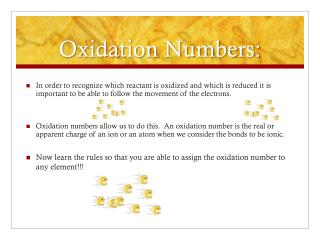DownloadDownload PresentationOxidation Numbers:

# Oxidation Numbers:

Télécharger la présentation## Oxidation Numbers:

- - - - - - - - - - - - - - - - - - - - - - - - - - - E N D - - - - - - - - - - - - - - - - - - - - - - - - - - -
##### Presentation Transcript

1. Oxidation Numbers: • In order to recognize which reactant is oxidized and which is reduced it is important to be able to follow the movement of the electrons. • Oxidation numbers allow us to do this. An oxidation number is the real or apparent charge of an ion or an atom when we consider the bonds to be ionic. • Now learn the rules so that you are able to assign the oxidation number to any element!!!

2. Oxidation Numbers: • Elements always have an oxidation number of zero. • K -K is 0 • N2 -N is 0 • Oxygen is always -2 unless it is in a peroxide and then it is -1. • HNO3 - O is -2 • H2O2 - O is -1 • The charge on a monatomic ion is the oxidation number. • Fe3+ is +3 • Br1- is -1 • Hydrogen is always +1 unless it is in a metal hydride and then it is -1. • HNO3 - H is +1 • CaH2 - H is -1

3. Determining Oxidation Numbers • K2CrO4 • O is -2 • K is +1 • Cr must be +6 • Cr2O3 • O is -2 • Cr must be +3 • Note that elements can have more than one oxidation number. • The sum of oxidation numbers in a neutral compound must be zero. • The sum of oxidation numbers in a polyatomic ions must equal the charge on the ion. • NO31- • O is -2 • N is +5

4. Examples: • H is +1 because the molecule is neutral, S must be -2 • O is -2, there are two O in the molecule therefore S must be +4 • O is -2, there are three O in the ion therefore S must be +4 to give an overall charge of 2- • Determine the oxidation number of sulfur in the following molecules: • H2S • SO2 • SO32-

5. Use of Oxidation Numbers: • In a redox reaction, one element loses electrons, while another gains them. Oxidation numbers allow you to keep track of these electron transfers and also allow you to determine whether a reaction is a redox reaction or not! • The following three reactions take place when coal with impurities of sulfur is burned. The result is the production of acid rain! • For each of these reactions determine the oxidation numbers of each element and then determine if the reaction is a redox reaction or not.

6. 1. • S + O2 SO2 • ___ ____ ____ ,_____ • Redox? _______ • 2. • 2SO2 + O2 2SO3 • ___ ,____ ____ ____ ,____ • Redox? ________

7. 3. • SO3 + H2O  H2SO4 • __, __ __ ,__ __, __, __ • Redox? _____ • 1. 0 0 +4 ,-2 Sulfur is oxidized, oxygen reducedREDOX • 2. +4, -2 0 +6, -2 Sulfur is oxidized, oxygen is reduced  REDOX. • 3. +6, -2 +1 , -2 +1, +6, -2 No change in the oxidation numbers of the elements therefore reaction number three is not a REDOX reaction.

8. Reducing – Oxidizing Agents • Mg(s) + S(s)  MgS(s) • 0 0 +2,-2 (oxidation numbers) • Reducing Agent • The substance that loses electrons. • Mg is oxidized in the previous reaction but it is the REDUCING AGENT. • Oxidation number increases. • Oxidizing Agent • The substance that gains electrons. • S is reduced in the previous reaction but it is the OXIDIZING AGENT. • Oxidation number decreases.

9. Lead contamination in drinking water can result in brain and nervous system damage. If tap water is slightly acidic it can dissolve the lead solder in pipes by the following reaction: • Pb(s) + 2H+(aq)  Pb2+(aq) + H2(g) • In this case the hydrogen ions from the acidic tap water oxidize the lead, while the lead reduces the hydrogen ions.

10. Reactivity of Metals • Metals (M) vary in their reactivity with acids (HA). • M(s) + 2HA(aq)  H2(g) + MA(aq) • The metal is oxidized, the hydrogen is reduced, the metal dissolves and hydrogen gas is produced. • The metal is considered to be the reducing agent. • Identify the most reactive and least reactive metal by examining the following picture;

11. Complex ions or neutral compounds may also be oxidizing and reducing agents. Consider the reaction that occurs when nitrogen dioxide gas bubbles in a solution containing silver ions. • Ag+(aq) + NO2(g) + H2O(l)  Ag(s) + NO3-(aq) +2H+(aq) • +1 +4,-2 +1, -2 0 +5, -2 +1 • Silver is reduced and nitrogen in the nitrogen dioxide is oxidized. • The oxidation numbers of oxygen and hydrogen do not change. • NO2 is the reducing agent. Silver ions are the oxidizing agent.

12. Use the oxidation numbers to identify the oxidizing and reducing agents in the following reactions: • A) CH4 + 2 O2 CO2 + 2H2O • B) Pb + H2SO4  H2 + PbSO4 • C) 2Mg + CO2  C + 2MgO • Oxidizing Agent Reducing Agent • A) O2 CH4 • B) H2SO4Pb • C) CO2 Mg

13. Chapter 20.2-Read pages 639  643 • Page 641 #9 & # 10 • http://www.youtube.com/watch?v=EHe8-AFMsMA • Page 643 #13  #16 • Worksheet Redox -2